Related Articles

# Find the first non-repeating character from a stream of characters

• Difficulty Level : Hard
• Last Updated : 09 Jun, 2021

Given a stream of characters, find the first non-repeating character from stream. You need to tell the first non-repeating character in O(1) time at any moment.

If we follow the first approach discussed here, then we need to store the stream so that we can traverse it one more time to find the first non-repeating character at any moment. If we use extended approach discussed in the same post, we need to go through the count array every time first non-repeating element is queried. We can find the first non-repeating character from the stream at any moment without traversing any array.

The idea is to use a DLL (Doubly Linked List) to efficiently get the first non-repeating character from a stream. The DLL contains all non-repeating characters in order, i.e., the head of DLL contains first non-repeating character, the second node contains the second non-repeating and so on.

We also maintain two arrays: one array is to maintain characters that are already visited two or more times, we call it repeated[], the other array is an array of pointers to linked list nodes, we call it inDLL[]. The size of both arrays is equal to alphabet size which is typically 256.

1. Create an empty DLL. Also create two arrays inDLL[] and repeated[] of size 256. inDLL is an array of pointers to DLL nodes. repeated[] is a boolean array, repeated[x] is true if x is repeated two or more times, otherwise false. inDLL[x] contains a pointer to a DLL node if character x is present in DLL, otherwise NULL.
2. Initialize all entries of inDLL[] as NULL and repeated[] as false.
3. To get the first non-repeating character, return character at head of DLL.
4. Following are steps to process a new character ‘x’ in a stream.
• If repeated[x] is true, ignore this character (x is already repeated two or more times in the stream)
• If repeated[x] is false and inDLL[x] is NULL (x is seen first time). Append x to DLL and store address of new DLL node in inDLL[x].
• If repeated[x] is false and inDLL[x] is not NULL (x is seen second time). Get DLL node of x using inDLL[x] and remove the node. Also, mark inDLL[x] as NULL and repeated[x] as true.

Note that appending a new node to DLL is O(1) operation if we maintain tail pointer. Removing a node from DLL is also O(1). So both operations, addition of new character and finding first non-repeating character take O(1) time.

Below image is a dry run of the above approach: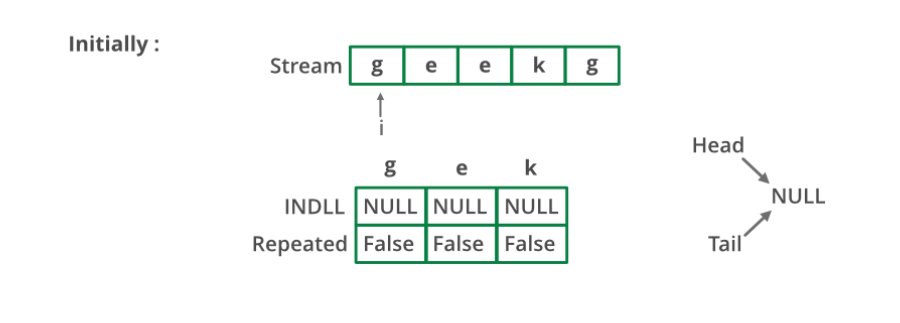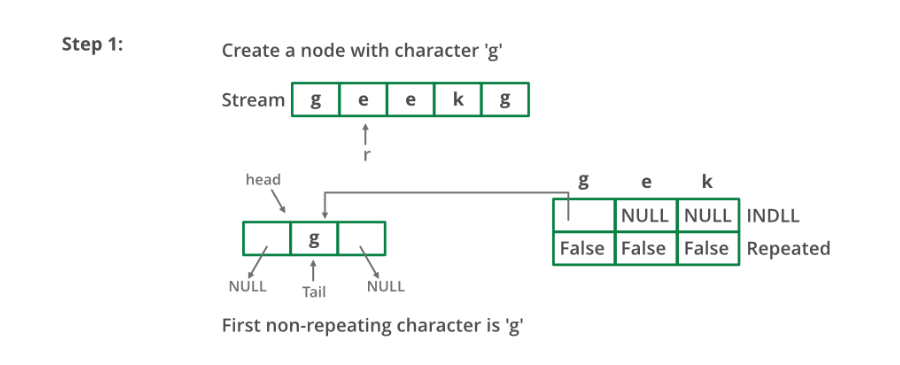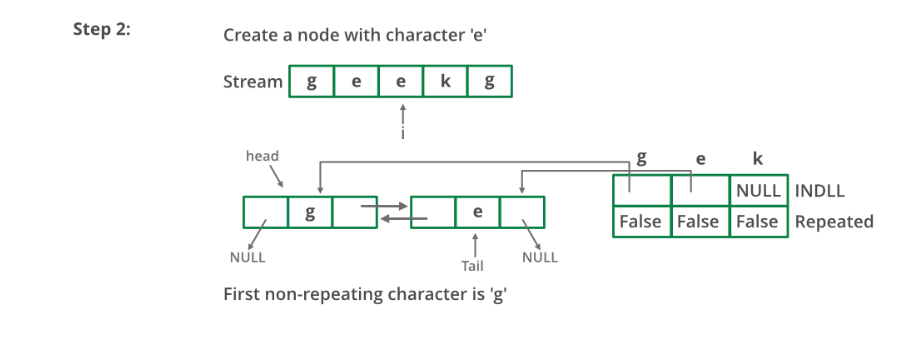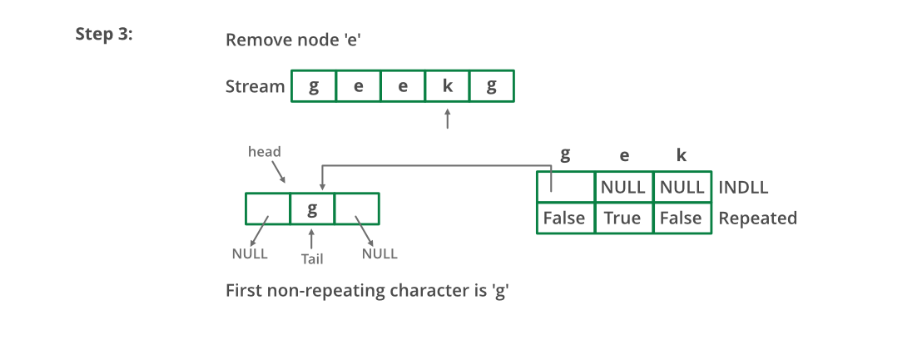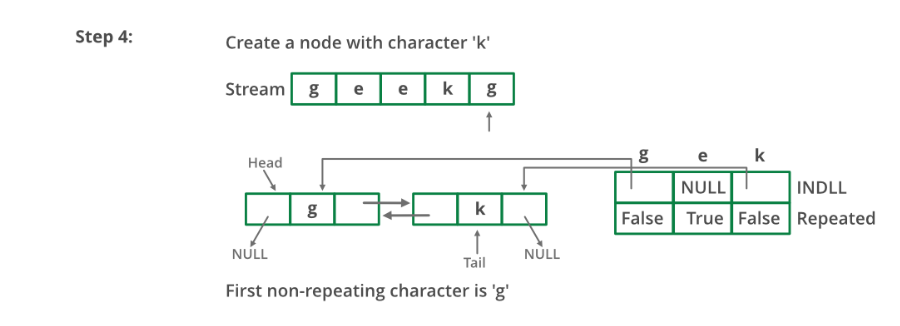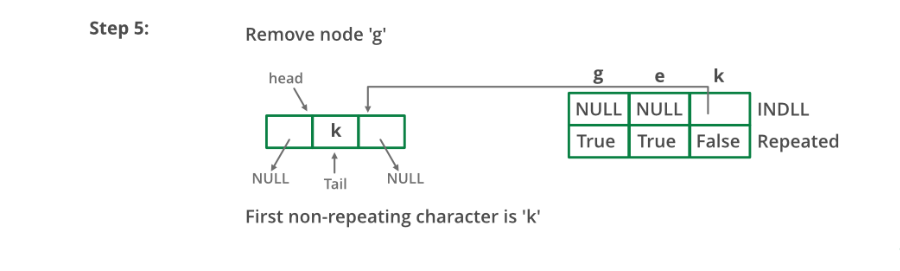Below is the implementation of the above approach:

## C++

 `// A C++ program to find first``// non-repeating character``// from a stream of characters``#include ``#define MAX_CHAR 256``using` `namespace` `std;` `// A linked list node``struct` `node {``    ``char` `a;``    ``struct` `node *next, *prev;``};` `// A utility function to append a character x at the end``// of DLL. Note that the function may change head and tail``// pointers, that is why pointers to these pointers are``// passed.``void` `appendNode(``struct` `node** head_ref,``                ``struct` `node** tail_ref, ``char` `x)``{``    ``struct` `node* temp = ``new` `node;``    ``temp->a = x;``    ``temp->prev = temp->next = NULL;` `    ``if` `(*head_ref == NULL) {``        ``*head_ref = *tail_ref = temp;``        ``return``;``    ``}``    ``(*tail_ref)->next = temp;``    ``temp->prev = *tail_ref;``    ``*tail_ref = temp;``}` `// A utility function to remove a node 'temp' fromt DLL.``// Note that the function may change head and tail pointers,``// that is why pointers to these pointers are passed.``void` `removeNode(``struct` `node** head_ref,``                ``struct` `node** tail_ref, ``struct` `node* temp)``{``    ``if` `(*head_ref == NULL)``        ``return``;` `    ``if` `(*head_ref == temp)``        ``*head_ref = (*head_ref)->next;``    ``if` `(*tail_ref == temp)``        ``*tail_ref = (*tail_ref)->prev;``    ``if` `(temp->next != NULL)``        ``temp->next->prev = temp->prev;``    ``if` `(temp->prev != NULL)``        ``temp->prev->next = temp->next;` `    ``delete` `(temp);``}` `void` `findFirstNonRepeating()``{``    ``// inDLL[x] contains pointer to``    ``// a DLL node if x is present``    ``// in DLL. If x is not present, then inDLL[x] is NULL``    ``struct` `node* inDLL[MAX_CHAR];` `    ``// repeated[x] is true if x is repeated two or more``    ``// times. If x is not seen so far or x is seen only``    ``// once. then repeated[x] is false``    ``bool` `repeated[MAX_CHAR];` `    ``// Initialize the above two arrays``    ``struct` `node *head = NULL, *tail = NULL;``    ``for` `(``int` `i = 0; i < MAX_CHAR; i++) {``        ``inDLL[i] = NULL;``        ``repeated[i] = ``false``;``    ``}` `    ``// Let us consider following stream and see the process``    ``char` `stream[] = ``"geeksforgeeksandgeeksquizfor"``;``    ``for` `(``int` `i = 0; stream[i]; i++) {``        ``char` `x = stream[i];``        ``cout << ``"Reading "` `<< x << ``" from stream \n"``;` `        ``// We process this character only if it has not``        ``// occurred or occurred only once. repeated[x] is``        ``// true if x is repeated twice or more.s``        ``if` `(!repeated[x]) {``            ``// If the character is not in DLL, then add this``            ``// at the end of DLL.``            ``if` `(inDLL[x] == NULL) {``                ``appendNode(&head, &tail, stream[i]);``                ``inDLL[x] = tail;``            ``}``            ``else` `// Otherwise remove this character from DLL``            ``{``                ``removeNode(&head, &tail, inDLL[x]);``                ``inDLL[x] = NULL;``                ``repeated[x]``                    ``= ``true``; ``// Also mark it as repeated``            ``}``        ``}` `        ``// Print the current first non-repeating character``        ``// from stream``        ``if` `(head != NULL)``            ``cout << ``"First non-repeating character so far "``                    ``"is "``                 ``<< head->a << endl;``    ``}``}` `/* Driver code */``int` `main()``{``    ``findFirstNonRepeating();``    ``return` `0;``}`

## Java

 `// A Java program to find first non-repeating character``// from a stream of characters` `import` `java.util.ArrayList;``import` `java.util.List;` `public` `class` `NonReapeatingC {``    ``final` `static` `int` `MAX_CHAR = ``256``;` `    ``static` `void` `findFirstNonRepeating()``    ``{``        ``// inDLL[x] contains pointer to a DLL node if x is``        ``// present in DLL. If x is not present, then``        ``// inDLL[x] is NULL``        ``List inDLL = ``new` `ArrayList();` `        ``// repeated[x] is true if x is repeated two or more``        ``// times. If x is not seen so far or x is seen only``        ``// once. then repeated[x] is false``        ``boolean``[] repeated = ``new` `boolean``[MAX_CHAR];` `        ``// Let us consider following stream and see the``        ``// process``        ``String stream = ``"geeksforgeeksandgeeksquizfor"``;``        ``for` `(``int` `i = ``0``; i < stream.length(); i++) {``            ``char` `x = stream.charAt(i);``            ``System.out.println(``"Reading "` `+ x``                               ``+ ``" from stream \n"``);` `            ``// We process this character only if it has not``            ``// occurred or occurred only once. repeated[x]``            ``// is true if x is repeated twice or more.s``            ``if` `(!repeated[x]) {``                ``// If the character is not in DLL, then add``                ``// this at the end of DLL.``                ``if` `(!(inDLL.contains(x))) {``                    ``inDLL.add(x);``                ``}``                ``else` `// Otherwise remove this character from``                     ``// DLL``                ``{``                    ``inDLL.remove((Character)x);``                    ``repeated[x]``                        ``= ``true``; ``// Also mark it as repeated``                ``}``            ``}` `            ``// Print the current first non-repeating``            ``// character from stream``            ``if` `(inDLL.size() != ``0``) {``                ``System.out.print(``                    ``"First non-repeating character so far is "``);``                ``System.out.println(inDLL.get(``0``));``            ``}``        ``}``    ``}` `    ``/* Driver code */``    ``public` `static` `void` `main(String[] args)``    ``{``        ``findFirstNonRepeating();``    ``}``}``// This code is contributed by Sumit Ghosh`

## Python3

 `# A Python program to find first non-repeating character from``# a stream of characters``MAX_CHAR ``=` `256` `def` `findFirstNonRepeating():` `    ``# inDLL[x] contains pointer to a DLL node if x is present``    ``# in DLL. If x is not present, then inDLL[x] is NULL``    ``inDLL ``=` `[] ``*` `MAX_CHAR` `    ``# repeated[x] is true if x is repeated two or more times.``    ``# If x is not seen so far or x is seen only once. then``    ``# repeated[x] is false``    ``repeated ``=` `[``False``] ``*` `MAX_CHAR` `    ``# Let us consider following stream and see the process``    ``stream ``=` `"geekforgeekandgeeksandquizfor"``    ``for` `i ``in` `range``(``len``(stream)):``        ``x ``=` `stream[i]``        ``print` `(``"Reading "` `+` `x ``+` `" from stream"``)` `        ``# We process this character only if it has not occurred``        ``# or occurred only once. repeated[x] is true if x is``        ``# repeated twice or more.s``        ``if` `not` `repeated[``ord``(x)]:` `            ``# If the character is not in DLL, then add this``            ``# at the end of DLL``            ``if` `not` `x ``in` `inDLL:``                ``inDLL.append(x)``            ``else``:``                ``inDLL.remove(x)``                ``repeated[``ord``(x)] ``=` `True` `        ``if` `len``(inDLL) !``=` `0``:``            ``print` `(``"First non-repeating character so far is "``)``            ``print` `(``str``(inDLL[``0``]))` `# Driver program``findFirstNonRepeating()` `# This code is contributed by BHAVYA JAIN`

## C#

 `// A C# program to find first non-repeating character``// from a stream of characters``using` `System;``using` `System.Collections.Generic;` `public` `class` `NonReapeatingC {``    ``readonly` `static` `int` `MAX_CHAR = 256;` `    ``static` `void` `findFirstNonRepeating()``    ``{``        ``// inDLL[x] contains pointer to a DLL node if x is present``        ``// in DLL. If x is not present, then inDLL[x] is NULL``        ``List<``char``> inDLL = ``new` `List<``char``>();` `        ``// repeated[x] is true if x is repeated two or more times.``        ``// If x is not seen so far or x is seen only once. then``        ``// repeated[x] is false``        ``bool``[] repeated = ``new` `bool``[MAX_CHAR];` `        ``// Let us consider following stream and see the process``        ``String stream = ``"geeksforgeeksandgeeksquizfor"``;``        ``for` `(``int` `i = 0; i < stream.Length; i++) {``            ``char` `x = stream[i];``            ``Console.WriteLine(``"Reading "` `+ x + ``" from stream \n"``);` `            ``// We process this character only if it has not occurred``            ``// or occurred only once. repeated[x] is true if x is``            ``// repeated twice or more.s``            ``if` `(!repeated[x]) {``                ``// If the character is not in DLL, then add this at``                ``// the end of DLL.``                ``if` `(!(inDLL.Contains(x))) {``                    ``inDLL.Add(x);``                ``}``                ``else` `// Otherwise remove this character from DLL``                ``{``                    ``inDLL.Remove((``char``)x);``                    ``repeated[x] = ``true``; ``// Also mark it as repeated``                ``}``            ``}` `            ``// Print the current first non-repeating character from``            ``// stream``            ``if` `(inDLL.Count != 0) {``                ``Console.Write(``"First non-repeating character so far is "``);``                ``Console.WriteLine(inDLL);``            ``}``        ``}``    ``}` `    ``/* Driver code*/``    ``public` `static` `void` `Main(String[] args)``    ``{``        ``findFirstNonRepeating();``    ``}``}` `// This code has been contributed by 29AjayKumar`

## Javascript

 ``

Output:

```Reading g from stream
First non-repeating character so far is g
First non-repeating character so far is g
First non-repeating character so far is g
First non-repeating character so far is g
First non-repeating character so far is g
First non-repeating character so far is g
First non-repeating character so far is g
First non-repeating character so far is g
First non-repeating character so far is k
First non-repeating character so far is k
First non-repeating character so far is k
First non-repeating character so far is s
First non-repeating character so far is f
First non-repeating character so far is f
First non-repeating character so far is f
First non-repeating character so far is f
First non-repeating character so far is f
First non-repeating character so far is f
First non-repeating character so far is f
First non-repeating character so far is f
First non-repeating character so far is f
First non-repeating character so far is f
First non-repeating character so far is f
First non-repeating character so far is f
First non-repeating character so far is f
First non-repeating character so far is o
First non-repeating character so far is r